# Previous Exams che31301 mass transfer spring 2011 quiz #1 I

 Page 1/2 Date conversion 20.05.2018 Size 125.86 Kb.
1   2

Previous Exams
CHE31301 MASS TRANSFER Spring 2011

QUIZ #1
I. A vertical pipe (25-mm ID), 2 m long, and closed at the bottom contains a 25-cm-deep layer of water at the bottom. The temperature and pressure of the surrounding gas (pure nitrogen) are 21oC and 760 mm Hg respectively. The vapor pressure of water at 21oC is 19 mm Hg. The nitrogen in contact with the open end of the pipe is dry.

1. The mass fraction of water vapor at the water surface is 0.016216

2. A. The mole fraction of water vapor at 1.0 m from the open end is independent of time.

B. The mole fraction of water vapor at the gas-liquid interface is independent of time.

 a. A and B are true b. Only A is true c. Only B is true d. A and B are false

3. A. If the surrounding nitrogen is saturated with water vapor, there will be no (net) evaporation.

B. It will take longer time for the water level to drop if the diameter is 50-mm ID, everything else remains the same.

 a. A and B are true b. Only A is true c. Only B is true d. A and B are false

4. A. The molar average velocity is constant within the pipe.

B. vA (A = water vapor) is constant within the pipe.

 a. A and B are true b. Only A is true c. Only B is true d. A and B are false

5. If the mole fraction of H2O is 0.1 then the mass fraction of H2O is 0.066667

II. Pulverized coal pellets, which may be approximated as carbon spheres of radius ro = 1 mm, are burned in a air (21% O2) at 1500 K and 1 atm. Oxygen is transferred to the particle surface by diffusion, where it is consumed in the reaction C + O2  CO2. The diffusivity DA for O2 is 1.810-4 m2/s. Assuming the surface reaction rate to be infinite and neglecting change in ro,

1. (5 pts) Determine the steady-state O2 molar consumption rate in kmol/s. (Show all your work) Gas constant Rg = 0.08206 m3atm/(kmolK)

3.86×10-9 kmol/s

II. Pulverized coal pellets, which may be approximated as carbon spheres of radius ro = 1 mm, are burned in a air (21% O2) at 1500oK and 1 atm. Oxygen is transferred to the particle surface by diffusion, where it is consumed in the reaction C + O2  CO2. The diffusivity DA for O2 is 1.810-4 m2/s. Assuming the surface reaction rate to be infinite and neglecting change in ro,

b) (5 pts) Determine the mole fraction of O2 at r = 3 mm. (Show all your work)

0.14

CHE31301 MASS TRANSFER Spring 2011

QUIZ #2

Note: Your answers must be correct to 3 significant figures and have the appropriate units.

I. A biofilm consists of living cells immobilized in a gelatinous matrix. A toxic organic solute (species A) diffuses into the biofilm and is degraded to harmless products by the cells within the biofilm. We want to treat 0.2 m3 per hour of wastewater containing 0.14 mole/m3 of the toxic substance phenol using a system consisting of biofilms on rotating disk as shown below.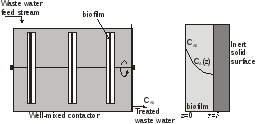Determine the required surface area of the biofilm with 1 mm thickness to reduce the phenol concentration in the outlet stream to 0.025 mole/m3. The rate of disappearance of phenol (species A) within the biofilm is described by the following equation
rA = − k1cA where k1 = 0.0024 s-1
The diffusivity of phenol in the biofilm at the process temperature of 25oC is 2.0×10-10 m2/s. Phenol is equally soluble in both water and the biofilm.
1) Determine the rate of phenol processed by the biofilms in mol/h: 0.0230 mol/h
2) The concentration of A in the biofilm is give by cA = B1sinh(mz) + B2cosh(mz), the numerical value of m is

3,464.1 m-1

For questions (3) and (4) use m = 3 mm-1, determine the numerical values with units for
3) B2 =  0.024876 mole/m3 4) B1 = 0.025 mole/m3

For the next question use cA = 0.025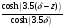where z is in mm and cA is in mole/m3

5) Determine the molar flux of A at z = 0 1.75×10-8 mol/m2s

6) Determine the molar flux of A at z = 0.5 mm 2.95×10-9 mol/m2s

II. In a hot combustion chamber, oxygen diffuses through a stagnant film of air with thickness L to the carbon surface where it reacts to make CO and CO2. The mole fraction of oxygen just outside the stagnant film (z = 0) is 0.21. The reaction may be assumed to be instantaneous. No reaction occurs in the gas film. The chamber is at 1 atm, 1000 K, and L = 10 cm. The diffusivity of oxygen at these conditions is 0.35 cm2/sec. The following reaction occurs at the carbon surface:
4C + 3O2  2CO + 2CO2

(7) Determine the molar flux of oxygen in mol/scm2 8.6610-8 mol/cm2s

(8) Determine the mole fraction of oxygen at z = 4 cm 0.12429
III.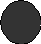membrane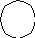Figure III. Conceptual model for a bioartificial organ.

Oxygen transport is of critical importance in the design of bioartificial organs. In one type of device, therapeutic cells such as the islets of Langerhans for the treatment of diabetes, are contained within a spherical membrane shown in Figure III. Let R be the distance from the center of the organ to the inside surface of the membrane, met be the tissue metabolic volumetric oxygen consumption rate (met is a constant, which is the rate of oxygen consumption per unit tissue volume), De be the diffusivity of oxygen within the tissue space, and  be the void fraction within the tissue space. The concentration of oxygen at the inside surface of the membrane is CA0.

9) The oxygen concentration, CA, within the tissue space can be obtained by solving the following differential equation

Ans: _________
A)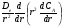= met B)= met
C)= met(1  ) (ANS.) D) None of the above.

10) The two boundary conditions required to solve the above equation are:

r = R, CA = CA0 r = 0,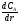= 0

CHE31301 MASS TRANSFER Spring 2011

QUIZ #3

Note: Your answers must be correct to 3 significant figures and have the appropriate units.

I. XI.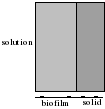The concentration of A within the biofilm is given by
CA = CAo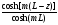, where m2 =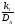k1 = first order rate constant = 1.5x10-4 /s

DA = diffusivity of A in the biofilm = 1.26x10-5 cm2/s

L = biofilm thickness = 0.2 cm

z = distance from the outer (solution) surface of the biofilm

CAo = 0.4 mol/liter

1) If the mass transfer resistance in the solution is negligible and the concentration of A in the biofilm is related to the concentration of A in the solution (CA) by the equilibrium relation CA = 0.4 CA , determine CA
CA = 1.0 mol/liter

2) If the surface area of the biofilm is As, the rate of substrate consumption within the biofilm is given by
A) k1CAAsL B) CAomAsDAtanh(mL) (ANS.)
C) k1CAoAsL D) None of the above.
II. Consider a spherical organism of radius R within which respiration occurs at a uniform volumetric rate of rA =  k1CA. That is, oxygen (species A) consumption is governed by a first-order, homogeneous chemical reaction. A molar concentration of CA(R) = CA,0 is maintained at the surface of the organism, and an expression for the radial distribution of oxygen, CA(r), within the organism is given by
CA(r) = CA,0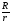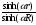Data: R = 0.16 mm, diffusion coefficient for oxygen transfer DAB = 10-8 m2/s, CA,0 = 610-5 kmol/m3, and k1 = 30 s-1.
3) The numerical value of is

5.48104 m-1
4) If = 5104 m-1, the concentration of oxygen at the center of the organism is 3.2210-7 kmol/m3

5) If = 5.0104 m-1, molar flux of A at r = R is 2.62×10-8 kmol/m2

6) If = 5.0104 m-1, molar flux of A at r = 0 is _____0_____
III. A solute diffuses through a membrane that separates two compartments A and B that have different initial concentrations. The solute concentrations in the two compartments as a function of time, CA and CB are shown in Figure III-1. The volumes of the two compartments are VA and VB.
(A) VA < VB (B) Solute diffuses from compartment B to A.
a. A and B are true b. Only A is true c. Only B is true d. A and B are false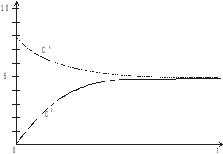1   2

The database is protected by copyright ©ininet.org 2016
send message

Main page